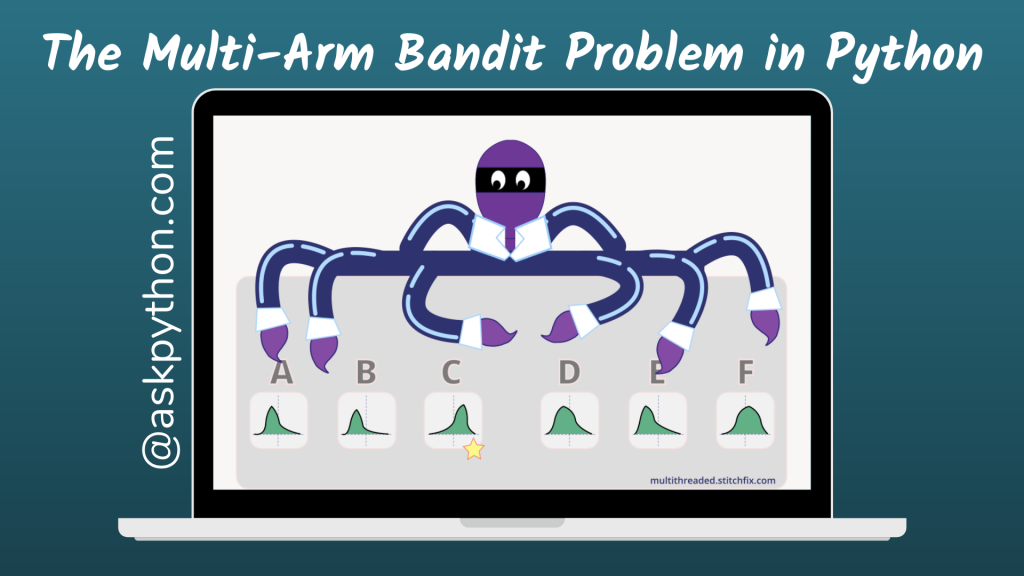# The Multi-Arm Bandit Problem in PythonThe n-arm bandit problem is a reinforcement learning problem in which the agent is given a slot machine with n bandits/arms. Each arm of a slot machine has a different chance of winning. Pulling any of the arms either rewards or punishes the agent, i.e., success or failure.

The agent’s goal is to pull the bandits/arms one at a time such that the total reward received after the operation is maximized. Furthermore, the issue description specifies that the agent does not know the likelihood of the weapons’ success. It eventually learns via trial and error, as well as value evaluation.

This tutorial will teach us how to utilize the policy gradient approach, which employs TensorFlow to build a basic neural network comprised of weights proportional to each of the available arms’ likelihood of obtaining the slot machine’s prize. In this strategy, the agent selects a machine arm based on an e-greedy approach.

It means that the agent will often pick the action with the highest anticipated value, but it will also choose at random.

In this manner, the spy tests out each of the several guns to understand more about them. When the agent acts, such as selecting an arm of the slot machine, it is rewarded with either a 1 or a -1.

## Implementing The Bandit Problem in Python

The following is a straightforward implementation of the n-arm/multi-arm bandit issue written in Python:

For our code implementation, we choose n=6 (6 arms of a slot machine) and their numbers are [2,0,0.2,-2,-1,0.8].

We will progressively discover that the agent learns and effectively selects the bandit with the highest payoff.

### Step 1 : Importing Modules

The method `tf.disable_v2_behavior` (as the name implies) disables any global behaviors that change between TensorFlow 1.x and 2.x and causes them to operate as intended for 1.x.

```import numpy as np
import tensorflow.compat.v1 as tf
tf.disable_v2_behavior()
```

### Step 2 : Computing Rewards for the Arms

We specify our bandits in a slot arms array. The length of the array is stored in `len_slot_arms`. The method discovers reward() creates a random integer with a mean of 0 from a normal distribution.

The lower the arm/bandit number, the more likely the agent will return a positive reward (1).

```slot_arms = [2,0,0.2,-2,-1,0.8]
len_slot_arms = len(slot_arms)
def findReward(arm):
result = np.random.randn(1)
if result > arm:
#returns a positive reward
return 1
else:
#returns a negative reward
return -1
```

### Step 3 : Setting up a Neural Agent

The TensorFlow library’s method `tf.rese_default_graph` clears the default graph stack and resets the global default graph. Lines 2 and 3 establish the weights of the specific bandits as 1 and then conduct the actual arm selection.

```tf.reset_default_graph()
weights = tf.Variable(tf.ones([len_slot_arms]))
chosen_action = tf.argmax(weights,0)
```

The training is handled by the code below. It initially feeds the network the reward and the specified action (arm). The loss is then computed by the neural network using the algorithm shown below. This loss is then used to improve network performance by updating the network.

```reward_holder = tf.placeholder(shape=,dtype=tf.float32)
action_holder = tf.placeholder(shape=,dtype=tf.int32)
responsible_weight = tf.slice(weights,action_holder,)
loss = -(tf.log(responsible_weight)*reward_holder)
update = optimizer.minimize(loss)

Loss = -log(weight for action)*A(Advantage from baseline(here it is 0)).
```

### Step 4: Training of Agent and finding the optimum arm/bandit

We train the agent by doing random activities and getting incentives. The code above starts a TensorFlow network, then a random action is chosen, and a reward is chosen from one of the arms. This incentive aids in network updates and is also displayed on the screen.

```total_episodes = 1000
total_reward = np.zeros(len_slot_arms) #output reward array
e = 0.1 #chance of taking a random action.
init = tf.initialize_all_variables()
with tf.Session() as sess:
sess.run(init)
i = 0

while i < total_episodes:
if np.random.rand(1) < e:
action = np.random.randint(len_slot_arms)
else:
action = sess.run(chosen_action)
reward = findReward(slot_arms[action])
_,resp,ww = sess.run([update,responsible_weight,weights], feed_dict={reward_holder:[reward],action_holder:[action]})
total_reward[action] += reward
if i % 50 == 0:
print ("Running reward for the n=6 arms of slot machine: " + str(total_reward))
i+=1

print ("The agent thinks bandit " + str(np.argmax(ww)+1) + " has highest probability of giving poistive reward")
if np.argmax(ww) == np.argmax(-np.array(slot_arms)):
print("which is right.")
else:
print("which is wrong.")
```

## Conclusion

Congratulations! You just learned how to solve the Multi-Arm Bandit Problem in the Python programming language. I hope you enjoyed it! 😇

Liked the tutorial? In any case, I would recommend you to have a look at the tutorials mentioned below:

Thank you for taking your time out! Hope you learned something new!! 😄